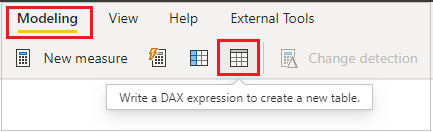Home » DAX » ROW DAX

# ROW DAXIn this blog, you will gain an understanding of how the “ROW” DAX function operates. This function falls within the “Table manipulation” function category.

The Row DAX function returns a table with a single row containing values that result from the expressions given to each column.

### Syntax

`ROW(<name>, <expression>, <name>, <expression>.....)`

### Parameters

name- The name given to the column, enclosed in double quotes.

expression- Any DAX expression that returns a single scalar value to populate. name.

#### Note

• Arguments must always come in pairs of name and expression.
• This function is not supported for use in Direct Query mode when used in calculated columns or row-level security (RLS) rules.

#### Return Value

The ROW function returns a single row table.

### Let’s get started-

Now, create a new table using a DAX expression by following these steps:

1. Navigate to the “Modeling” tab.
2. Choose the option labeled “Write a DAX expression to create a new table.Create table

After that write below DAX code-

```Table = ROW("Total Sales", SUM(Orders[Sales]),

"Total Profit", SUM(Orders[Profit]),

"Total Discount", SUM(Orders[Discount]))```

This function will generate a single-row table containing the total sale, profit, and discount values. Refer to the result displayed in the screenshot below for visualization.Row DAX Function

Refer DAX post:- DAX Tutorials